# JILT 1997 (2) - van Noortwijk & De Mulder

## The Similarities of Text Documents

Kees van Noortwijk and Prof Richard V. De Mulder
Erasmus University
VanNoortwijk@rrj.frg.eur.nl DeMulder@rrj.frg.eur.nl

 Abstract 1. Conceptual information retrieval 1.1 Introduction 1.2 Implementing a system 1.3 Finding similar documents 2. Document similarity 2.1 Different forms 2.2 Calculating similarity 2.2.1 'Hits' and 'misses' 2.2.2 Weight 2.2.3 Amount of information 3. Calculating a similarity score 3.1 Formulae 3.2 Using information 3.3 For all documents 4. Conclusion ReferencesDownload

### Abstract

This article examines the possibility of calculating the similarity of text documents. This can be a first step in creating conceptual legal information retrieval systems, which can retrieve documents that resemble a certain example document given by the user. The method for calculating document similarity described here, which has been developed by the Centre for Computers and Law of the Erasmus University Rotterdam, is based on word use in documents.

Keywords: conceptual legal information retrieval, legal documents, word use.

This is a refereed article published on 30 June 1997.

Citation: Van Noortwijk K and De Mulder R, 'The Similarities of Text Documents', 1997 (2) The Journal of Information, Law and Technology (JILT). <http://elj.warwick.ac.uk/jilt/artifint/97_2noor/>. New citation as at 1/1/04: <http://www2.warwick.ac.uk/fac/soc/law/elj/jilt/1997_2/noortwijk/>

### 1. Conceptual information retrieval based on the word use in documents

#### 1.1 Introduction

Electronic data retrieval systems are among the most frequently used computer applications in the field of law. Given that fact, it is surprising how little these systems have changed in the past thirty years. In almost every legal database, Boolean searching using words within the documents to be looked up is still the only retrieval method that is supported. This means that a lawyer who wants to look up certain legal cases has to make a crucial conversion. They know what the cases should be about; so they have to speculate which words should be present in such cases. The set of words must also be assembled with great care. Common words should be avoided because they will probably be present in irrelevant documents as well. Very specific terms carry a danger too because it is possible that only a synonym is used in a relevant document. In addition to this, many users find it difficult to use the logical operators necessary for combining words in a search request. Therefore, assembling and applying effective search requests is still a specialist job. As regards the output of a search request, a problem is that in many cases the documents found are not ordered according to their (expected) relevance. This means that users have little information to go on as regards relevance when browsing through this list.

Not surprisingly, many users - not only in the legal field - have problems with this relatively complicated and inefficient procedure for retrieving information. Computer scientists have proposed various improvements for the 'classic' information retrieval systems. One of the most interesting ideas is that 'conceptual' retrieval systems could be constructed. In such systems, information that conforms to certain concepts can be looked up. The formulation of these concepts is based on knowledge about the field (in this case, the law). Wildemast and De Mulder (1992) have given an overview of the attempts that have been made to build such systems.

#### 1.2 Implementing a conceptual retrieval system

In the literature, several methods have already been proposed to make the implementation of a conceptual retrieval system possible. In this article we will concentrate on a method for improving the search operation itself (Wildemast and De Mulder; 1992). The system of which a certain part is described makes it possible for users to define their own concepts, and uses these concepts to optimise the recall and precision of a search operation. Concepts that have been defined and applied can be more precisely re-defined, for instance on the basis of the results of search operations. Furthermore, a concept can be stored for later re-use. In this way the systems can 'learn': the knowledge about a certain legal concept that has been built up during a retrieval operation can be put to use again for later search requests.

The crucial point of course is how the user can define a legal concept that is to be used for retrieving documents (De Mulder et al 1993). As the purpose of a concept in this case is to specify a certain set of documents, a possible way of defining it is also in terms of documents: the concept should include a certain document, but exclude another. This is the method we have chosen in our system: the user has to give examples of the documents to be retrieved. Documents that are identified as being relevant to a concept are called exemplars. Consequently, the searching facility of the system will search for documents that are similar to the exemplars. In order to fulfil this task, the program will compare properties or attributes of potentially relevant documents with those of the exemplars. In theory, all kinds of attributes could be used here, ranging from simple syntactical characteristics of the documents to facts that could only be unveiled by analysing the documents at a semantic level. At this stage, we have concentrated on word use in the documents, which includes the words used in these documents as well as their frequency. An important reason for this choice is that these characteristics can be measured automatically for all documents in a database without difficulty.

#### 1.3 Finding similar documents

After the user has specified one or more exemplars, the system has to search the database and present a list of documents, ordered according to how similar these documents are to the exemplars. In this article we will concentrate on the very first step in this process. This is to find the document that is most similar to a given exemplar, or has the highest average similarity to a given set of exemplars in a database. To achieve this, a similarity measure has to be defined, which makes it possible to quantify exactly how similar two document in a database are. This similarity measure is discussed in the next section. An important characteristic of this measure is that to a certain extent it is based on word use in the whole database. This means that when two documents are compared, word use in all other documents that are part of the same database is taken into account as well. Given a database, it is possible to calculate similarities for all possible document pairs from that database, although the number of combinations can, of course, become very large as the size of the database increases.

The similarity between two documents forms a possible first step in the conceptual retrieval process. The process can be continued by other techniques, especially by introducing counter exemplars in addition to the exemplars. This can be useful in order to 'teach' the system the finesses of the concept the user has in mind. For more information on this, see Wildemast and De Mulder (1992). In this article, we will concentrate on the exemplars and on finding the documents that match these as closely as possible.

### 2. Document similarity 

#### 2.1 Different forms of similarity

Two objects, in this case documents, can be similar in several ways. For instance, we could focus on

1. the typesetting used in the documents;
2. the general layout (of pages, headings, etc.);
3. the number of word tokens (occurrences) and word types (different words) present in each document;
4. the word types themselves, and their frequency;
5. the average/maximum/minimum length of words, sentences, paragraphs or pages;
6. the language used in the documents;
8. the subject they are on;
9. the effect they have (for instance when they are published or read),

The ordering of these alternatives is not a coincidence. It ranges from pure similarities in the form (1, 2, 3, 4, 5), via similarities of mixed signature (6, 7), to similarities at the semantic (8) or pragmatic level (9). Here, we will concentrate on similarities at the form level and especially on the type of similarity mentioned under 4 (word use and word frequency) although the similarity mentioned under 3. (number of word tokens and word types) will play a role in a way as well. Therefore, when we speak of document similarity, from now on we mean the following:

Similarity is the degree in which two documents resemble each other, with respect to the form they have and especially with respect to the word types they contain.

In the past, similarity has played a role in quite a number of research projects. Many of these were carried out with the general aim of improving the structure or the usability of database systems. The research by Salton within the framework of the SMART-project can be mentioned here. In the overview report 'The SMART System' (Salton; 1971) he describes a method of forming clusters of documents within a database, which has certain characteristics in common (Salton ; 1971, p. 223 and Salton 1989). However, a drawback of the way this method is implemented is that only index words (added to each document) play a role in calculating similarity, which makes it impossible to classify documents completely automatically.

A method to select sets of documents with high similarity from a database is described by Smeaton and Van Rijsbergen (1981). The authors introduce an algorithm to speed up a search operation in a database. First a base document is chosen. From that point on only those documents which, based on the words they contain, could in theory be similar to the base document are taken into account. A method that has more or less the same purpose is described by Willett (1981). Unfortunately a drawback of both these methods is that they can only be used when the similarity of documents is calculated from the number of common word types, not when (as in our approach) different weights are associated with every word type.

Batagelj and Bren (1993) have recently compared a number of similarity measures. The authors not only define similarity, but also dissimilarity between objects, and use this measure to relate the different similarity measures known from literature to each other (Batagelj and Bren; 1993, p. 8-16). Some of their measures will be examined in the next section. We describe below our chosen method for calculating similarity.

#### 2.2 Calculating similarity: some basic concepts

Here the characteristic which forms the basis for calculating the similarity of two documents is the presence or absence of word types. For practical reasons, we will not take into account the frequency of a word type within each document (although the method could be extended to include this as well). The only type of frequency that plays a role here is the number of documents in which a word type appears. This characteristic, for which we will use the term document frequency, is strongly related to the dispersal of word types throughout the documents.

#### 2.2.1 'Hits' and 'misses'

When we want to determine the similarity of two documents by means of the word types present in these documents, two situations appear at first to be possible:

• a word type is present in both documents; because this means that the documents have a common characteristic, it should increase similarity. For this situation the term hit is used.
• a word type is present in one document, but not in the other; at this point the documents differ from each other and therefore similarity should decrease. This situation is called a miss.

It may be crucial (especially for misses) which document is used as a base document, when the documents are not of equal size (which would be quite a coincidence). Consider the following situation. We compare two documents, of which one contains 100 word types and the other 200. 50 hits are found (50 word types are present in both documents), which means that the number of misses is 50 when we look at it from the first document, and 150 when we take the second. We may conclude that comparing documents has a relative aspect, it depends on which document is taken as a basis. This means that it will not usually be sufficient to compare a document X with a document Y, but that it will also be necessary to compare Y with X.

When only the 'misses' are considered, another improvement is possible: instead of one we could count two types of misses. With two documents X and Y there could be

• a type 1 miss (in short, 'miss1') if a word type is present in document X, but not in Y; and
• a 'type 2 miss' (in short, 'miss2') if a word type is present in document Y, but not in X.

With these three characteristics, the number of hits, miss1s and miss2s, the relationship between two documents can be effectively established. However, when the documents do not stand on their own but are part of a database containing many other documents, there is a fourth characteristic. The other documents will probably contain a number of word types which are present neither in document X nor in Y. The absence of such a word type in both documents can even be considered to be a point of resemblance, which should increase the similarity of the documents. Therefore, this is also a kind of 'hit', just as in the situation where a word type is present in both documents. This means that next to two types of misses, two types of hits are also possible:

• a 'type 1 hit' (in short, 'hit1') if a word type is present in both documents; and
• a 'type 2 hit' (in short, 'hit2') if a word type is absent in both documents, but is used in other parts of the database.

The four situations which are discerned here (two types of hits and 2 types of misses) have also been mentioned in the literature. Hits and misses are often referred to by the letters a, b, c and d. A usually stands for the number of hit1s, b and c for the number of miss1s and miss2s and d for the number of hit2's .(Batagelj and Bren;1993)

#### 2.2.2 The 'weight' of a hit or miss

Not every word type is used the same number of times in a database. This does not necessarily influence the similarity of documents because, as stated earlier, in our definition word frequency within a document is not taken into account when calculating similarity. However, when the number of documents in which a word type is present (the 'document frequency' of a word type  ) differs from the number of documents in which other word types appear, this can have an influence on similarity. For the probability that a word type with a high (document) frequency is present in a certain pair of documents, and therefore is responsible for a 'hit1', is much higher than the probability that this happens with a low frequency word. Conversely, the probability of a 'hit2' is higher with word types of a low frequency. For the two types of misses an analogue conclusion can be drawn. This may be expressed in tabular form:

Table 1 The relationship between the frequency and the probability of hits and misses

 Probability for: Frequency of word types: High Low Hit1 high low Hit2 low high Miss1 low high Miss2 high low

The probability that a word type will cause a hit1, hit2, miss1 or miss2 is therefore directly related to the (document) frequency of that word type. That means that not every hit or miss can be considered to be of equal significance. When a word type with a frequency of only 2 (when the number of documents is high, say 20000) is found in a pair of documents, this gives us much more information than when a high frequency word type (for instance 'the', 'it', etc.) is found, and the similarity of the documents should therefore increase more in the first situation than in the second. This means that we cannot just count the number of hits and misses when calculating similarity. With every hit and miss, we have to correct for the probability that the hit or miss occurs in a certain database. This can be done by calculating two separate probabilities for every word type:

1. the probability of finding a word type in a certain document; this probability is of importance in case of a hit1 or a miss2, because in those cases the word type is present in the document to which we are comparing our base document.
2. the probability of missing a word type in a certain document; this probability has to be taken into account for a hit2 or a miss1, because in those cases the word type is absent in the document to which we are comparing our base document.

These probabilities only have to be calculated once for every word type. After that, when calculating the similarity of every pair of documents we can re-use these numbers again and again. The first probability is equal to the (document) frequency of the word type divided by the number of documents in the corpus. The second probability is equal to the difference between the number of documents and the (document) frequency of the word type, divided by the number of documents in the corpus. In a formula: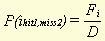(1)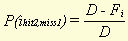(2)

Where Fi stands for the frequency of word type i (i.e. the number of documents in which word type i appears) and D for the number of documents in the whole database. Strictly speaking, in calculating these probabilities we have not taken into account that the base document (document X) is itself part of the database. To rectify that, some of the values in the two formulae (for instance, D in both denominators) would have to be decreased by 1. We have chosen not to do that, however. An important reason for this is that the formulae now constitute a symmetric model. The probability of finding a word type that appears in every document is equal to the probability of missing a word type that appears in no document (both probabilities are equal to 1), whereas the probability of finding a word type that appears in no document is equal to the probability of missing a word type that appears in every document (both equal to 0). This makes it easier to perform calculations, while the effect of the omission is minor, at least when the number of documents is sufficiently high.

While the probability of finding, for instance, a hit1 is high for those word types that appear in many documents, it is of course true that the significance of such a hit1 for document similarity in general is low. To compensate for this, it is therefore necessary to multiply every hit or miss with a 'weight factor' W, which should have a value opposite to that of the probability for the word. Two possible ways to calculate this weight factor would be to take the complement or the reciprocal of the probability:(3)(4)

A disadvantage of the second method, however, is that the weight factor would have a range of [1..¥], which could lead to very high values for the similarity scores calculated by means of these weights. Zero probabilities also present a problem because they would cause a division by zero. For these reasons the first method has been used to calculate hit and miss weights.

Using these weight factors, which have to be calculated for every word type, we can determine the adjusted number of hit1s, hit2s, miss1s and miss2s. These numbers equal the sum of all weights of the word types involved. For instance, the adjusted number of hit1s is equal to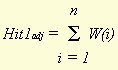(5)

where n stands for the number of hit1's, which is the number of word types that appear in both documents. These adjusted numbers of hits and misses are suitable for calculating similarity because they take into account which word types are 'responsible' for a specific hit or miss.

To make it possible to compare the similarity that is calculated by means of these adjusted numbers of hits and misses for different pairs of documents, the numbers have to be made relative to their respective maximum values. These maximum values are different for every document. They constitute the sum of all hit1 and miss1 weights of the word types present in the document, and the sum of all hit2 and miss2 weights of the word types not present in the document (but present in one of the other documents of the database). When we divide the adjusted numbers of hits and misses by these maximum values and multiply the result by 100%, we get hit and miss percentages. When similarity is calculated from these percentages, we get similarity values that are comparable for all possible pairs of documents from the database.

#### 2.2.3 The amount of information in every hit and miss

As stated in the previous section, the amount of information connected with a certain event is inversely proportional to the probability that the event will happen. For calculating similarity, we have used this fact to give every hit and miss the proper 'weight'. However, information theory gives us a method to quantify the amount of information connected with an event, and express this amount in a number, the number of bits. A bit (binary digit) is the smallest amount of information that can be measured. It is the amount of information we get from the answer to a yes/no question, when the probability for each answer is equal. The amount of information in bits that we get from an event can be derived from the probability that the event will happen, using the following formula: (De Mulder;1984, p 22)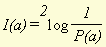(6)

I(a), the amount of information we get from event a, is equal to the 2-log of the reciprocal of the probability of a. With this formula, we can calculate the amount of information we get when we find a hit or miss in a pair of documents. The use of the logarithm to an extent compensates for the disadvantage of the use of the reciprocal of a probability that we have already mentioned (namely its high value for low frequency word types). Of course the amount of information calculated by means of (6) in general will be parallel to the respective (adjusted) number of hits or misses, but especially for low frequency word types it can be a useful tool to calculate similarity more precisely. Furthermore, it can also be used to compare the amount of information in documents and even in complete databases.

Of course the amount of information of a hit or miss can also be expressed as a percentage of the maximum possible amount in a document, as described in the previous section. This makes it possible to compare this characteristic for different pairs of documents.

### 3. Calculating a similarity score

#### 3.1 Formulae for calculating similarity

By means of the characteristics described in the previous sections, a similarity score for every pair of documents in a database can be calculated. Several formulae can be used for this, a number of which will be presented in this section. An explanation will be given as to which formula yields the best results and why that is the case.

When two documents are compared, and the numbers of hit1s, hit2s, miss1s and miss2s are determined, we have two figures which express how much the documents have in common (the number of hit1s and hit2s) and two which express how different they are (the number of miss1s and miss2s). The value of each of these figures can be compensated for their respective probabilities , and converted to a hit or miss percentage by dividing by the maximum possible value for a certain document. These percentages will be used here. Furthermore, we see it as essential that, in a similarity score, hits (at least one of the two types) should play a role because otherwise (if only misses are used) a dissimilarity score would result.

If we consult relevant literature with this in mind, we find a number of possible ways for calculating a similarity score. Batagelj and Bren (1993) mention no less than 22 different similarity and dissimilarity measures, from more than 15 different sources. However, all these measures are intended for calculating similarity based on characteristics of equal weight. As this is not the case here, none of these 22 similarity measures can be used right away. Some of them can be altered, however, because they are based on the principle that hits and misses should be converted into a percentage. These include  :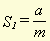(7)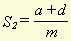(8)(9)

a, b, c and d stand for hit1, miss1, miss2 and hit2, respectively. m, in this case, stands for the total number of word types in the document. These formulae yield a type of similarity percentage because of the division by m and, therefore, can be compared for different pairs of documents. We can alter these formulae to make it possible to use the adjusted numbers of hits and misses, instead of just the absolute numbers. The result is then:(10)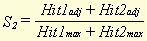(11)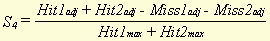(12)

In principle, each of these formulae could be used to calculate document similarity. The characteristics of each formula include the following

• In formula (10) similarity is equal to the hit1 percentage. It calculates the amount of 'overlap' between the two documents (range: [0..1]). This can be useful if we want to concentrate on common points in the documents, rather than taking into account the differences. A disadvantage remains that words that are missing from both documents (hit2s) are not taken into account at all. This can lead to biased results, especially when one of the documents is much larger than the other. A final problem could be that in almost every pair of documents there will at least be some overlap, because many word types are present in almost every document  . This would make it necessary to introduce a threshold value for similarity (for instance, only if similarity is greater than 0.5 should it be considered significant).
• In formula (11), hit1s as well as hit2s (and therefore all common points of the documents) are taken into account. It also has a range of [0..1]. Note that the outcome of (11) does not correspond to the average of the hit1 and hit2 percentages, except when both maximum values (Hit1max and Hit2max) are equal. That is only the case when a document contains exactly half of the adjusted number of word types in the whole database. It can be expected that similarity, calculated by means of this formula, for very large or small documents will be considerably lower than would follow from just the two hit percentages. A justification for this is that in these documents the relationship between the number of hit1s and the number of hit2s will be very biased as well. Still, a disadvantage of the formula could be that in a small document the hit2 percentage could play a dominant role (hit1s are of almost no influence at all) whereas in large documents the same could be true for the hit1 percentage.
• In formula (12) all the available characteristics are used. The total hit percentage from (11) is reduced by the total miss percentage. It is questionable whether this yields a correct result in this case. The reason for this doubt is that the number of misses is already represented in the hit1 and hit2 percentages. For the calculation of the maximum values Hit1max and Hit2max, all word types present in a document are taken into account. Some of these will become 'involved' in a hit1, the others will be miss1s. The hit1 percentage is thus already reduced by every miss1. Subtracting the miss percentage again could lead to a situation where misses play a more important role than hits. This is undesirable because we want to calculate similarity, not dissimilarity. Therefore, formula (12) does not offer a suitable solution in this instance.

This leads to the following conclusions:

• There are many ways to calculate similarity. However, the three ways presented here have in common the fact that they can be used when characteristics of different weight are involved. With most other similarity measures mentioned in the literature this is not possible.
• Formula (12), in which misses play a role, probably yields incorrect results. This is because the number of misses is already taken into account when calculating hit1 and hit2 percentages.
• Of the remaining two formulae, (11) has the advantage that hit2s, which can be very important for the similarity of documents, are taken into account.
• Formula (10), which calculates overlap between documents, could be useful when documents are of very different sizes. When using this formula, it is probably necessary to determine a threshold value for similarity in order to neutralise the low 'basic' similarity that almost every pair has.

#### 3.2 Using information instead of weight

As described in section 2.2.3, it is possible to calculate the amount of information of every hit and miss and use this as an alternative to the 'weight' of word types. It is possible to calculate similarity by means of these amounts of information. Especially when low probabilities are involved, this can lead to different similarity values because of the logarithmic nature of the information measure. For hit1s, this is the case with rare word types, and for hit2s with very common word types.

Using information instead of weight means that word types with low probabilities will have a stronger influence on the similarity score. Documents which both contain a rare word type, or which both miss a common word type, will get a higher similarity score. This means that attention shifts towards those characteristics that are most specific to a document. In a large database this could lead to a more reliable similarity score.

When we want to use hit information instead of weight, similarity can be calculated as follows: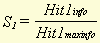(13)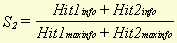(14)

#### 3.3 Calculating similarity for all documents in a database

Using the formulae described in the previous sections, it is possible to calculate the similarity between a single (example) document and all the other documents in the database. The similarity scores can be ordered, after which it becomes clear which documents most resemble the example document. The following procedure can be used:

1. All documents are read in; for every document a list of the word types that are present in it is compiled.
2. From the lists of word types per document a word list for the whole database is compiled. In this list an indication is also given of the number of documents in which a certain word type appears (the 'document frequency' of the word type).
3. Now the weight as well as the amount of information of a word type can be calculated, for the situation that the word type would be involved in a hit1 as well as in a hit2. These figures are registered in the type list, with the respective word type.
4. Using these weight and amounts of information, the maximum (adjusted) number of hit1s and hit2s and the maximum amount of hit1 and hit2 information can be calculated for every document (Hit1max, Hit2max, Hit1maxinfo and Hit1maxinfo). These figures are registered in the list of documents.
5. Then follows the most time consuming part of the process: the list of word types of the example document is compared to the lists of all other documents and, based on the lists, the (adjusted) number of hit1s and hit2s is calculated as well as the amount of information connected with these. From these, the similarity score for that particular document pair can be calculated. Note that because of the similarity measure chosen it is not necessary to calculate the number of misses.
6. Finally, a report is compiled in which all documents appear, ordered according to their similarity to the example document. If desired, the report can also state the characteristics (adjusted number of hits, and amount of information) which form the basis for every similarity score.

This technique has already been implemented in a computer program, and has been used to calculate similarity between the documents of a large statute law database (18803 documents, 143156 word types). In our experience it is possible, even in such a large database, to calculate the similarity of all documents with a given example document within minutes, using a normal (Pentium) PC. This proves that in principle the technique could be used in database search programs.

### 4. Conclusion

This article has discussed a specific set of techniques that can be used to build a conceptual information retrieval system. In the system proposed here, users can give examples of documents they want to retrieve from the database. When (at least) one example has been given, a first step is to let the system look for documents that most resemble the example given.

The method to determine the similarity of two documents introduced here can be used to achieve this. It is based on word use in all documents in a database. Several different formulae to calculate the similarity have been discussed. A computer program which makes use of one of these methods in general yields a list in which all documents are ordered according to their similarity to the example document. This list will assist users to find other good examples of what they are looking for.

Although the number of calculations necessary to determine the similarity scores is high, modern personal computers are powerful enough to accomplish this task within a reasonable time, even when the number of documents is large.

##### References

Batagelj V and Bren M (1993), Comparing Similarity Measures, University of Ljubljana, Ljubljana 1993.

De Mulder R.V. (1984), Een model voor juridische informatica(A Model for the application of computer science to law). With a summary in English, Vermande, Lelystad 1984.

De Mulder R.V, Van den Hoven M.J. and Wildemast C (1993), "The concept of concept in 'conceptual legal information retrieval'". 8th BILETA Conference Pre-proceedings, p. 79-92. CTI Law Technology Centre, University of Warwick, Warwick 1993.

Salton (ed) (1971), The SMART retrieval system, experiments in automatic docu-ment processing, Prentice-Hall, Englewood Cliffs N.J. 1971.

Salton (1989), Salton G, Automatic Text Processing; The transformation, Analysis, and Retrieval of Information by Computer, Addison-Wesley Publishing Company, Reading Massachusetts, 1989.

Smeaton A.F. and C.J. van Rijsbergen (1981), 'The Nearest Neighbour Problem in Information Retrieval', Proceedings of the Fourth International Conference on Information Storage and Retrieval, p. 83-87. ACM, New York 1981.

Van Noortwijk C (1995), Het woordgebruik meester. Een vergelijking van enkele kwantitatieve aspecten van het woordgebruik in juridische en algemeen Nederlandse teksten (Legal word use, a comparison of some quantitative aspects of the word use in legal and general Dutch texts). With a summary in English, Koninklijke Vermande, Lelystad 1995.

Wildemast, C.A.M. and R.V. De Mulder (1992), Some design Considerations for a Conceptual Legal Information Retrieval System, Grütters, C.A.F.M., J.A.P.J. Breuker, H.J. Van den Herik, A.H.J. Schmidt, C.N.J. de Vey Mestdagh (eds), Legal Knowledge Based Systems: Information Technology & Law, JURIX'92, Koninklijke Vermande, Lelystad, Nl, 1992, pp.81-92.

Willett P, (1981), "A Fast Procedure for the Calculation of Similarity Coeffi-cients in Automatic Classification", Information Processing and Management, Volume 17, p. 53-60. Pergamon Press, Oxford 1981.

#### Footnotes

 The contents of this and the following section are partly based on a chapter from Van Noortwijk (1995)

 As this 'document frequency' is the only frequency considered here, from now on we will refer to it simply as 'frequency'.

 For the moment, we will only use weights to achieve this. Later on in this section, the amount of information will be used as well.

 Batagelj and Bren (1993), p. 8. As sources for these formulae they mention, without giving a complete reference: S1: (Russel and Rao 1940); S2: (Kendall, Sokal-Michener 1958); S4: (Hamann 1961).

 Word types found in every document are of no importance to similarity, because they have a weight that equals 0.